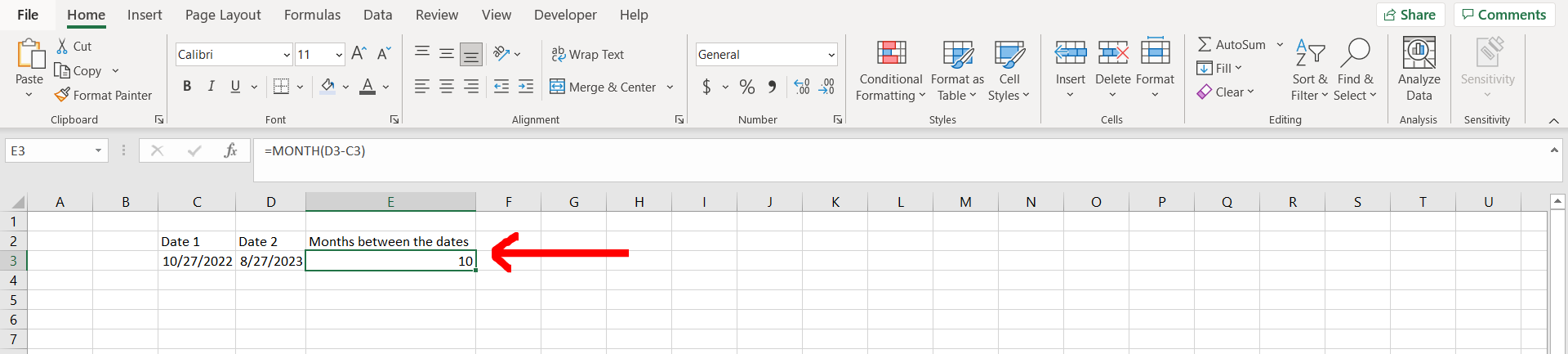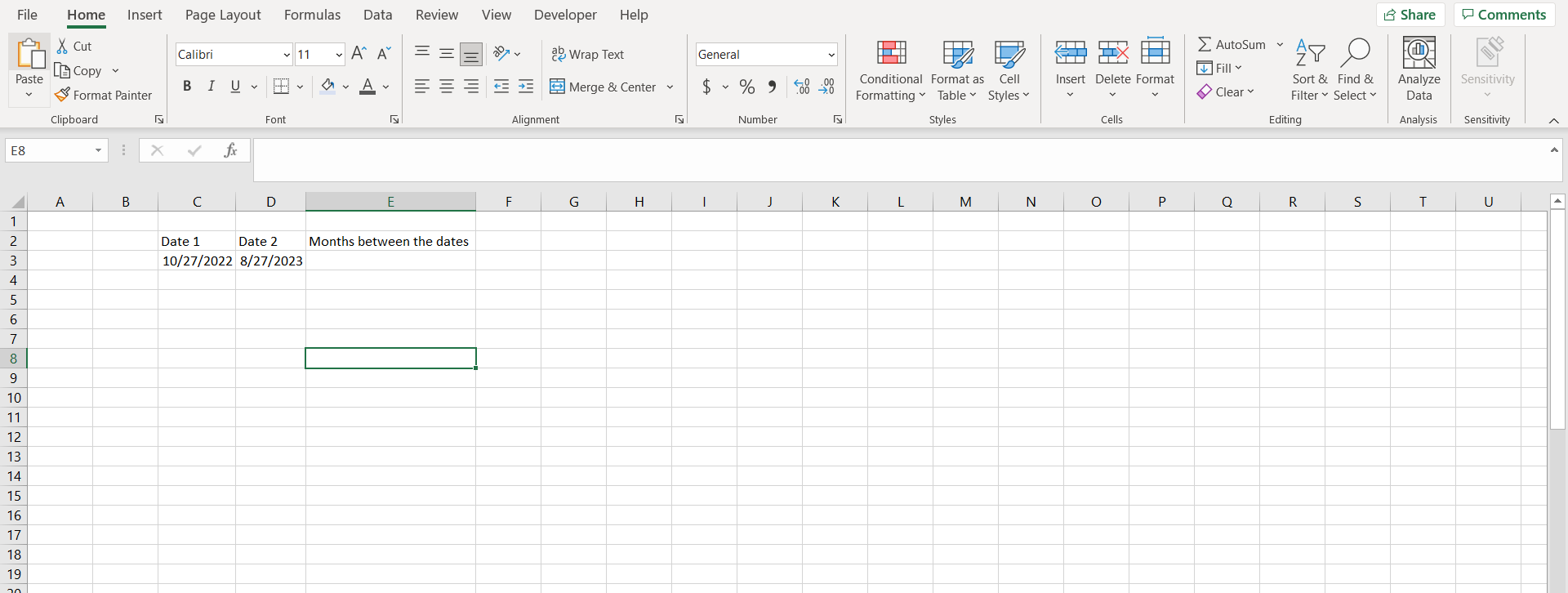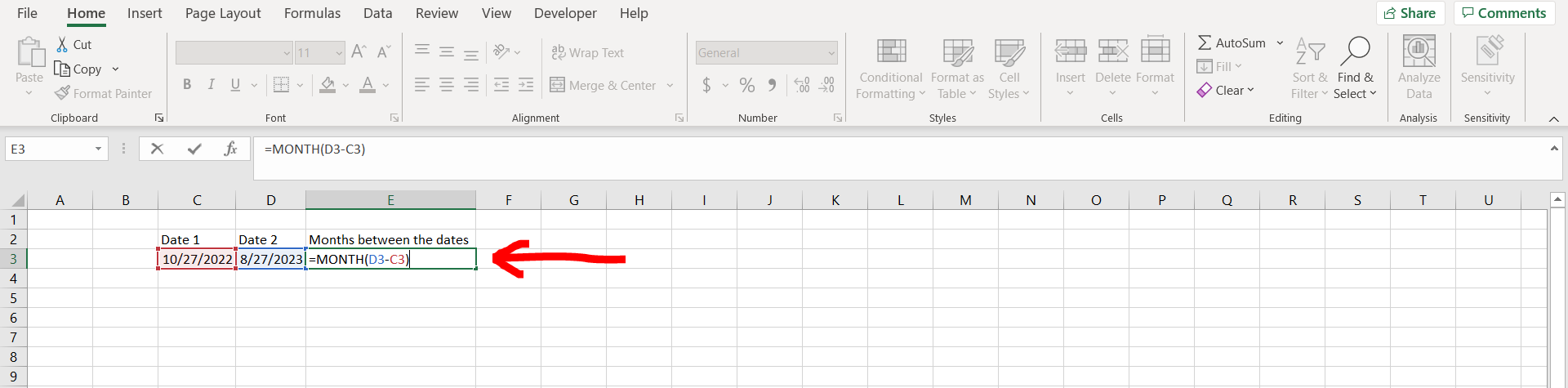# How many months between two dates in excelExcel offers a very interesting way to find the months between 2 dates. We can cater to this problem statement by using the “MONTH” formula. We can perform the below mentioned way to find the months between 2 dates in excel:

### How many months between two dates excel:Step-1: Excel workbook with 2 dates– Open the desired Excel workbook having 2 dates which needs to be subtracted to find the months between them

### Step-2: Apply the formula– Now type “=MONTH(<DATE1>-<DATE2>)”. This will tell excel that we want to subtract the 2 dates present in the cells, and then convert the result into number of months.

### Final Image : Getting the result– Now press “Enter” on your keyboard to get the number of months between these 2 dates as shown in the image above.